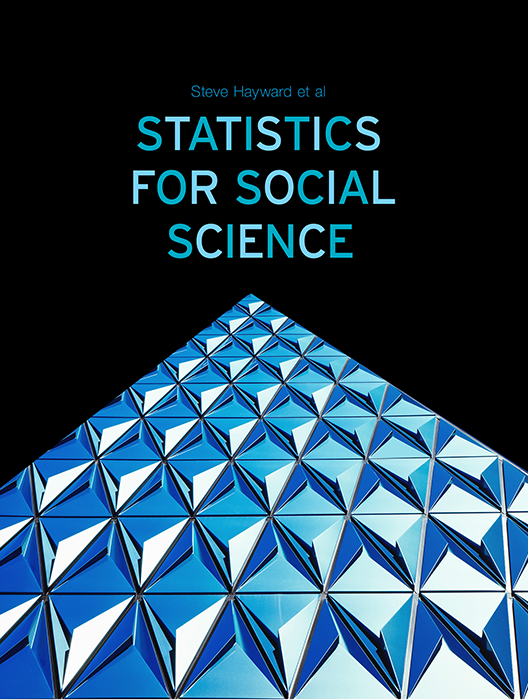Statistics for Social Science

Lead Author(s): Stephen Hayward

Statistics for Social Science takes a fresh approach to the introductory class. With learning check questions, embedded videos and interactive simulations, students engage in active learning as they read. An emphasis on real-world and academic applications help ground the concepts presented. Designed for students taking an introductory statistics course in psychology, sociology or any other social science discipline.

This content has been used by 8,525 students

What is a Top Hat Textbook?

Top Hat has reimagined the textbook – one that is designed to improve student readership through interactivity, is updated by a community of collaborating professors with the newest information, and accessed online from anywhere, at anytime.

• Top Hat Textbooks are built full of embedded videos, interactive timelines, charts, graphs, and video lessons from the authors themselves
• High-quality and affordable, at a significant fraction in cost vs traditional publisher textbooks

Key features in this textbook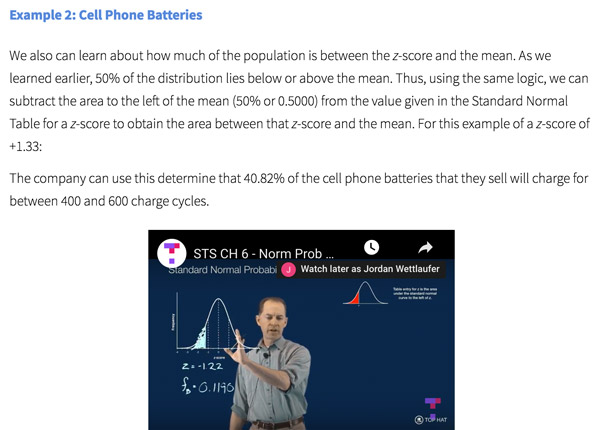Our Statistics for Social Science textbook allows students to manipulate data, visualize the effects discussed, and explore Lightboard videos that feature instructor explanations to reinforce concepts and calculations.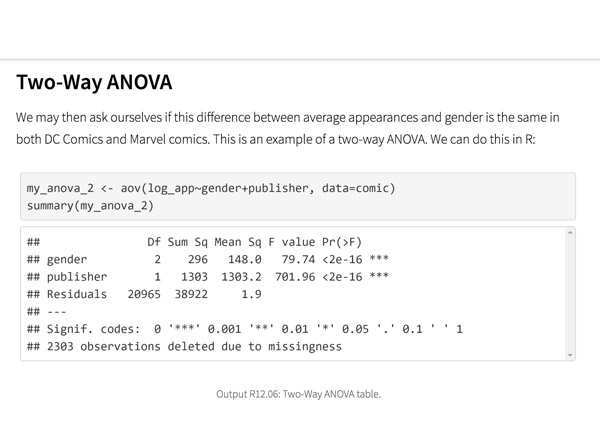Top Hat’s interactive offering includes a complementary module on using R software for data management, graphics, and conducting statistical analyses with examples and practice questions.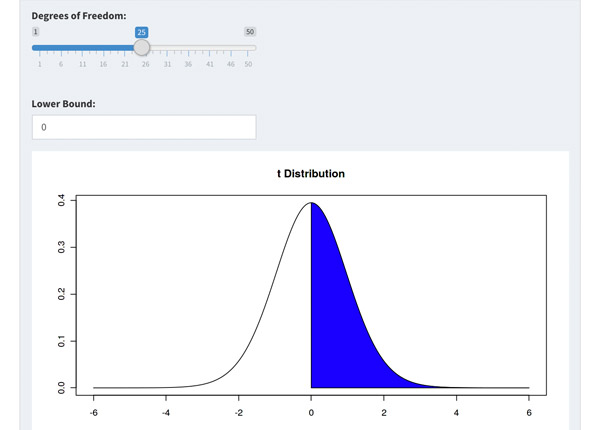Built-in assessment questions embedded throughout chapters so students can read a little, do a little, and test themselves to see what they know!

Comparison of Social Sciences Textbooks

Consider adding Top Hat’s Statistics for Social Sciences textbook to your upcoming course. We’ve put together a textbook comparison to make it easy for you in your upcoming evaluation.

Top Hat

Steve Hayward et al., Statistics for Social Sciences, Only one edition needed

Pearson

Agresti, Statistical Methods for the Social Sciences, 5th Edition

Cengage

Gravetter et al., Essentials of Statistics for The Behavioral Sciences, 9th Edition

Sage

Gregory Privitera, Essentials Statistics for the Behavioral Sciences, 2nd Edition

Pricing

Average price of textbook across most common format

Up to40-60%more affordable

Lifetime access on any device

$200.83 Hardcover print text only $239.95

Hardcover print text only

$92 Hardcover print text only Always up-to-date content, constantly revised by community of professors Content meets standard for Introduction to Anatomy & Physiology course, and is updated with the latest content In-Book Interactivity Includes embedded multi-media files and integrated software to enhance visual presentation of concepts directly in textbook Only available with supplementary resources at additional cost Only available with supplementary resources at additional cost Only available with supplementary resources at additional cost Customizable Ability to revise, adjust and adapt content to meet needs of course and instructor All-in-one Platform Access to additional questions, test banks, and slides available within one platform Pricing Average price of textbook across most common format Top Hat Steve Hayward et al., Statistics for Social Sciences, Only one edition needed Up to40-60%more affordable Lifetime access on any device Pearson Agresti, Statistical Methods for the Social Sciences, 5th Edition $200.83

Hardcover print text only

Pearson

Gravetter et al., Essentials of Statistics for The Behavioral Sciences, 9th Edition

$239.95 Hardcover print text only Sage McConnell, Brue, Flynn, Principles of Microeconomics, 7th Edition $92

Hardcover print text only

Always up-to-date content, constantly revised by community of professors

Constantly revised and updated by a community of professors with the latest content

Top Hat

Steve Hayward et al., Statistics for Social Sciences, Only one edition needed

Pearson

Agresti, Statistical Methods for the Social Sciences, 5th Edition

Pearson

Gravetter et al., Essentials of Statistics for The Behavioral Sciences, 9th Edition

Sage

Gregory Privitera, Essentials Statistics for the Behavioral Sciences, 2nd Edition

In-book Interactivity

Includes embedded multi-media files and integrated software to enhance visual presentation of concepts directly in textbook

Top Hat

Steve Hayward et al., Statistics for Social Sciences, Only one edition needed

Pearson

Agresti, Statistical Methods for the Social Sciences, 5th Edition

Pearson

Gravetter et al., Essentials of Statistics for The Behavioral Sciences, 9th Edition

Sage

Gregory Privitera, Essentials Statistics for the Behavioral Sciences, 2nd Edition

Customizable

Ability to revise, adjust and adapt content to meet needs of course and instructor

Top Hat

Steve Hayward et al., Statistics for Social Sciences, Only one edition needed

Pearson

Agresti, Statistical Methods for the Social Sciences, 5th Edition

Pearson

Gravetter et al., Essentials of Statistics for The Behavioral Sciences, 9th Edition

Sage

Gregory Privitera, Essentials Statistics for the Behavioral Sciences, 2nd Edition

All-in-one Platform

Access to additional questions, test banks, and slides available within one platform

Top Hat

Steve Hayward et al., Statistics for Social Sciences, Only one edition needed

Pearson

Agresti, Statistical Methods for the Social Sciences, 5th Edition

Pearson

Gravetter et al., Essentials of Statistics for The Behavioral Sciences, 9th Edition

Sage

Gregory Privitera, Essentials Statistics for the Behavioral Sciences, 2nd Edition

Steve HaywardRio Salado College

A lifelong learner, Steve focused on statistics and research methodology during his graduate training at the University of New Mexico. He later founded and served as CEO of Center for Performance Technology, providing instructional design and training development support to larger client organizations throughout the United States. Steve is presently lead faculty member for statistics at Rio Salado College in Tempe, Arizona.

Joseph F. Crivello, PhDUniversity of Connecticut

Joseph Crivello has taught Anatomy & Physiology for over 34 years, and is currently a Teaching Fellow and Premedical Advisor of the HMMI/Hemsley Summer Teaching Institute.

Explore this textbook

Read the fully unlocked textbook below, and if you’re interested in learning more, get in touch to see how you can use this textbook in your course today.

ANOVA and the F-DistributionEstimating the population of elusive animals such as the jaguar can be very difficult. In order to predict how the population of these species will evolve, scientists need to have a way to estimate the ratio of males to females. Recently, scientists have developed a method to accomplish this by measuring the size of the footprints left by the jaguars. How do we know that the results of such a test are statistically significant? An analysis of variance (ANOVA) test can show us that the difference between the mean sizes of footprints in males and females is enough to estimate gender! 

Chapter Objectives

After completing this chapter, you will be able to:

• Describe characteristics of the F-distribution
• Determine when ANOVA testing is appropriate
• Explain the rationale and steps involved in computing the F-statistic
• Compute degrees of freedom and determine the value of critical F
• Perform a two-group and three-group one-factor between-groups Analysis of Variance (ANOVA)
• Perform and interpret a post-hoc test for a one-factor, three-level between-groups ANOVA
• Explain and interpret a one-factor within-groups ANOVA
• Explain the rationale for a two-factor ANOVA
• Report and interpret results of ANOVA tests

Introduction

Previously, you learned about the z-test and t-test for single samples, followed by the t-test for two samples. The F-test can be used to compare two or more samples. Recall that the t-statistic is equal to the difference between two sample means (x̄1- x̄2) divided by a measure of variability—the standard error of the difference between means—to yield a one number statistic, the t-value. The probability or likelihood by chance of the computed t-score was then determined from the t-distribution. The t-test formula does not allow for a third group mean. For comparing two samples, the t-test and the F-test yield identical outcomes. However, in order to compare three or more samples, it is necessary to use the F-test, which compares sample variances to assess group differences, instead of the t-test, which compares sample means; the t-test formula is restricted to only two means.

Comparing sample variances takes a different approach to evaluating group differences. The total variance in the dependent scores is partitioned into two components: within-groups variance and between-groups variance. Within-groups variance is an estimate of individual differences and non-systematic or confounding factors. Between-groups variance is an estimate of the effect of the independent variable, individual differences, and non-systematic confounding factors. Figure 12.1 illustrates hypothetical between-group variance in the spending habits of online shoppers, mall shoppers, and television home-shopping channel shoppers. As you can see in the figure, there is quite a bit of intra-group or within-group variability in spending patterns as well. A statistical test is needed to determine whether there are statistically significant differences in the amount of money spent per month by different types of shoppers.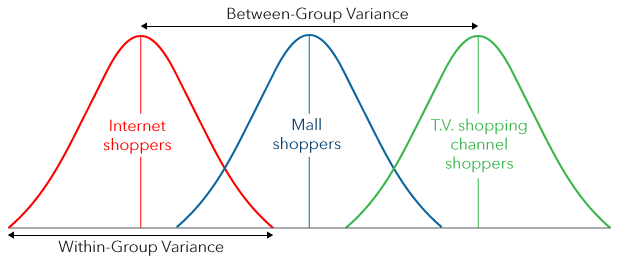Figure 12.1: ​Hypothetical distributions illustrating within-groups and between-groups variance

The F-Distribution

The F-distribution is similar to the t-distribution in that it is a family of distributions, where the shape and percent of the area beyond a critical score are dependent upon degrees of freedom. Unlike the t-family of distributions, The F-family of distributions is one-sided; there are no negative F-scores. Also, there are two sets of degrees of freedom (df). The first pertains to the number of group comparisons or levels of the independent variable (df numerator). The second pertains to the number of participants or scores in the dataset (df denominator). The F-distribution is the comparison sampling distribution for Analysis of Variance (ANOVA).

Why Not Compute Multiple t-tests?

You might be tempted to compute a series of t-tests when comparing three samples (i.e., Group 1 vs. Group 2, Group 1 vs. Group 3, and Group 2 vs. Group 3). However, doing so would be very cumbersome and would greatly increase the likelihood of Type I errors. Let’s briefly review the relationship between probability and Type I errors. When the α level is set at .05, there is a 5% chance of making a Type I error. The probability of a Type I error is the same for every comparison made on the same data set. In other words, the probability of making a Type I error when comparing Group 1 to Group 2 is 5% and likewise for the Group 1 to Group 3 comparison and the Group 2 to Group 3 comparison. If multiple test statistics are computed on the same data set, the probability of Type I errors would be 5% for each comparison.

Probability Pyramiding

The issue with this method is that conducting multiple comparisons on the same data set without adjusting the probability level leads to Probability Pyramiding. Conducting multiple t-tests on the same data set would lead to a greatly increased effective Type I error probability level. It would be as if the alpha level was increased exponentially.

For example, if two independent analyses such as two t-tests are carried out with α set to .05, the probability that at least one analysis will result in a Type I error is greater than 5 percent. In order to determine the new probability level, a researcher should calculate the probability that a significant result would not be obtained in either of the two tries and then subtract this from one. Thus,

P(Type I error) = 1 - (1-α) (1-α) = 1 - (.95) (.95) = 1 - (.95)2 = 1 - .902 = .098.

The probability of making a Type I error in this case is .098 and not .05!

Example

Suppose you wanted to compare the motor speed of left-handed people, right-handed-people and ambidextrous people and you set the α level for your study at .05. The independent variable is the handedness group, with three levels. The dependent variable is motor speed. If the t-test were the only statistic available to you, the following comparisons would need to be made. See Figure 12.3 below: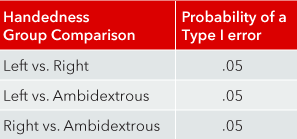Figure 12.3: Probability of a Type I error​

​Following the method above for determining the actual probability that any one of the pairwise comparisons would be spurious or statistically significant by chance, i.e., the probability of a Type I error, gives us the following:

P(Type I error) = 1 - (1-α) (1-α) (1-α) = 1 - (.95) (.95) (.95) = 1 - (.95)3 = 1 - .857 = .143.

The probability of mistakenly concluding there is a significant effect in at least one of the comparisons is now a whopping 14% instead of just 5%!

Each individual pair-wise analysis increases the likelihood of probability pyramiding. Fortunately, Analysis of Variance takes a different approach to multiple group comparisons, which eliminates the Type I error probability pyramiding problem.

Assumptions of the ANOVA Model

Before we learn how to compute the F-statistic, let’s discuss when the F-statistic is appropriate to use for group comparisons. The assumptions of random sampling and/or random assignment are the same for ANOVA as for other parametric, inferential statistics (e.g. z-tests, t-tests, and correlations). Likewise, since the comparison distribution is normal, the distribution of each sample should be approximately normal. In addition, the variances of each sample should be approximately equal; this is frequently referred to as the homogeneity of variance assumption.

Parametric Statistics: A set of statistical procedures which assumes that the population in question is approximately normally distributed and conforms to particular sets of parameters.

Inferential Statistics: A branch of statistics that involves analyzing sample data with the purpose of inferring population parameters.

Why Homogeneity of Variance Matters

In the formulas for computing the F-statistic, the variances of each group in a study are added together. To estimate how differences between groups compare to differences within any given group, there is a mathematical assumption that the variances of each group are approximately equal. The figure below depicts four different sample distributions. The green group may be considered the control group or baseline measure. The red, yellow, and blue groups represent potential treatment effects. Note that the red and yellow distributions are more spread out, that is, they have larger within-group variances, than the blue distribution. The variance components of the F-statistic will be disproportionately affected not only by the mean differences, but also by the differences in variance for each of the groups. Violations of homogeneity of variance can lead to biased findings. In other words, if the assumptions of a statistical test are violated, then the outcome of the statistical test is not reliable.Figure 12.4: Four distributions with unequal variances

When to Use an F-test (ANOVA)Figure 12.5: Assumptions of the ANOVA model​​

The F-statistic

The F-statistic is a ratio of two variances and therefore is often referred to as the F-ratio. The numerator or top portion of the ratio represents the variability between the groups being compared. In a typical between-groups research design, participants assigned to different levels of the independent variable are treated differently. Therefore, differences in the participants’ dependent scores are a function of how they are treated in the study and any individual differences among the participants in each treatment group.

The denominator or lower portion of the ratio represents the variability within groups. Since all of the participants within any one level of the independent variable are treated the same, any differences in the dependent scores reflect only individual differences among the participants.

Example of Between-Groups Versus Within-Groups Variance

Let’s use a practical example to think critically about the composition of between-groups and within-groups variance. In 2007, Maier, Moller, Friedman, and Meinhardt tested the effect of color on performance on anagrams. In the first study, participants were briefly exposed to red, green, or black colored pieces of paper immediately prior to completing an anagram task. The independent variable was color, with three levels: red, green, or black. The dependent variable was the number of anagrams solved correctly in five minutes.

Since there are three groups in the study design, the t-test is not appropriate: the F-statistic is the appropriate test statistic. The within-group variability consists of the difference in anagram performance scores within a given color group: red, green, or black. Logically speaking, we would expect that since the participants in any given color group received the same exact manipulation or level of the independent variable, any variability in scores would be due to individual differences in anagram performance rather than due to the color manipulation. This measure of variability is known as the within-groups variability and is nearly identical to the standard error of the differences between means computed for the t-test.

The variability between groups is influenced by two phenomena: the variability due to the color manipulation and variability due to individual differences. In order to evaluate the effect of the color manipulation, the F-statistic is a ratio of between-groups variance divided by within-groups variance.

The between-groups variance is often referred to as the treatment effect since the term reflects changes in scores due to the manipulation of the independent variable. The within-groups variance is often referred to as the error variance or error term. Any variability within groups is a measure of individual differences or confounding variables, not an effect of the independent variable.

In Maier, Moller, Friedman, and Meinhardt’s (2007) study example: Upon solving for F, we are left with a measure of the effect of the independent variable, color.

In the F-test, the between-groups variance is the estimated proportion of variance that is due to the independent variable. It is an estimate of the variance based on the differences among the means. Within-groups variance is an estimate of the variance within each of the samples.

Between-Groups Variance: reflects changes in the dependent variable that are due to systematic differences in the ways the groups were treated or selected. These changes include the effects of the independent variable and any confounding variables. This term is often referred to as the treatment variance since it reflects the variance due to the independent variable manipulation.

Within-Groups Variance: reflects changes in the dependent variable that are due to individual differences and/or other uncontrolled factors. This term is often referred to as the error variance or error term since it reflects variance due to uncontrolled factors.

12.01

In a study comparing two samples, either the t-test or the F-test would be appropriate.

A

True

B

False

12.02

The F-statistic is a ratio score resulting in a measure of the estimated proportion of variance due to the independent variable.

A

True

B

False

12.03

The term ‘homogeneity of variance’ means that the samples have unequal variances.

A

True

B

False

Using the F-table

As with z-scores and t-scores, you will need to look up the critical value of F in a table. To do so, you will need to know the following:

• The α level of the study
• The number of samples or groups being compared from the research design
• The degrees of freedom between groups and the degrees of freedom within groups

Computing Degrees of Freedom for a Between-Groups Design

To compute the degrees of freedom between groups, take the number of groups (or levels of the independent variable, often denoted as k) and subtract one. The degrees of freedom within-groups (written as dfw) is equal to the number of participants in the study (Ntotal) minus the number of groups (k).

Looking up Critical F

In order to determine the critical value of F, we need to look up the appropriate α level and appropriate degrees of freedom. Below is an excerpt from an F-table. Look down one column of values in the F-table. As the degrees of freedom within-groups increases, the critical value of F increases. Just as with the t-family of distributions, the smaller the sample size (as indicated by the degrees of freedom within-groups) the flatter and more spread out the F-distribution becomes, and the larger the critical F-value becomes—hence the use of an F-family of distributions instead of one standard normal curve.

How to use this table:

The following table gives critical values of F at the p = .05 level.

1. Obtain your F-ratio.  This has (x,y) degrees of freedom associated with it.
2. Go along x columns and down y rows.  The point of intersection is your F-ratio.
3. If your obtained value of F is equal to or larger than this critical F-value, then your result is statistically significant at that level of probability.

Example

A researcher obtains an F-ratio of 3.96 with (2, 24) degrees of freedom.  She goes across 2 columns and down 24 rows.  The critical value is 3.40.  The obtained F-ratio is larger than this value, so she concludes that the obtained F-ratio is likely to occur by chance less than 5% of the time, i.e., with p < .05.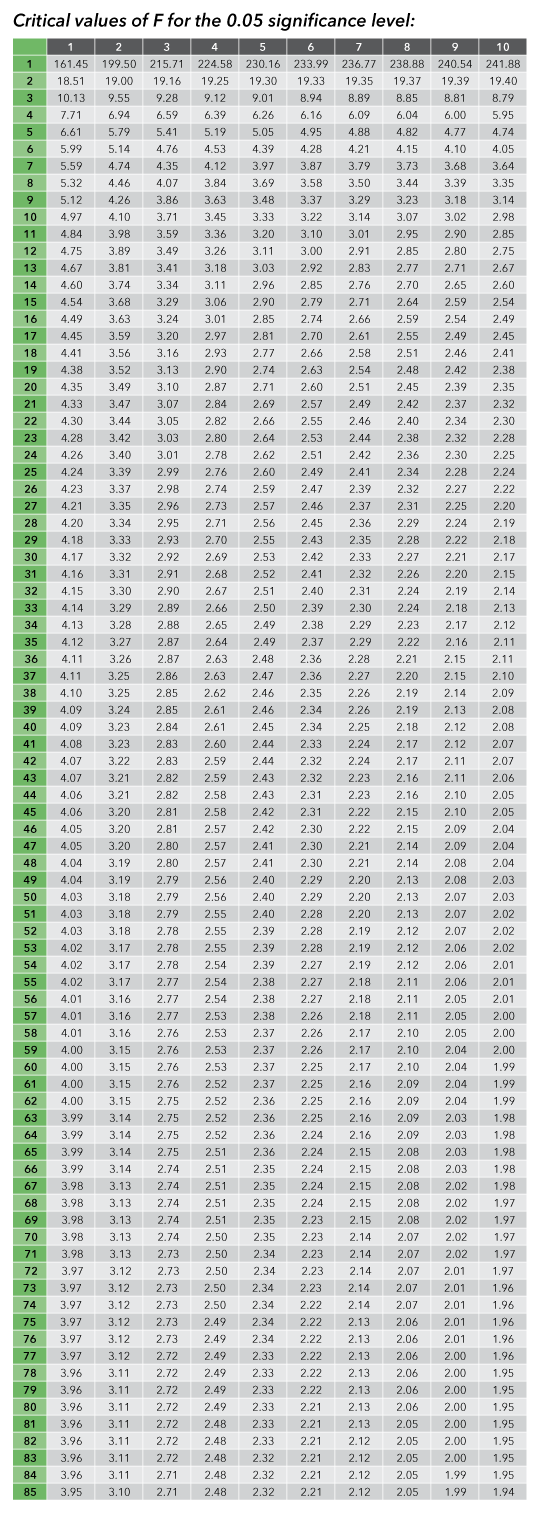Figure 12.6. F-table for use with ANOVA

Follow the first row across to locate the between-groups degrees of freedom. Then, move down the column to the appropriate within-groups degrees of freedom. The value in the cell is the critical value of F. For example, if the degrees of freedom between-groups was 2 and the degrees of freedom within-groups was 20, the critical F value would = 3.49.

Example: Degrees of Freedom Computation and Using the F-table

In our example from the Maier, Moller, Friedman, and Meinhardt (2007) color study, there are three groups or three levels of the independent variable: red, green, or black. Therefore, the degrees of freedom between groups (written as dfB) is:

dfBetween = 3-1

dfBetween = 2

The sample sizes in the Maier, Moller, Friedman, and Meinhardt (2007) study were as follows:

The df for the numerator is dfbetween, which is 2. The df for the denominator is dfwithin, which is 68. Starting at the upper-left hand corner of the table, go over two columns and down to 68 degrees of freedom. Note that the critical value of F = 3.13.

Let’s practice computing degrees of freedom and determining critical values of F from the table.

Read the research descriptions below and specify either the degrees of freedom between groups or the degrees of freedom within groups.

12.04

A total of 160 participants were assigned to a new medication, a standard medication, a placebo, or a no medication condition. What is the $df_{between}$?

12.05

Scientists investigated the effect of movie type on mood induction. Twenty people per film group watched a horror film, a romantic comedy, or a documentary, followed by the completion of a mood survey. What is the $df_{within}$?

12.06

Please click on the correct critical F value in the table, for the specified df. Use an α=.05.

$df_{between}$=2 $df_{within}$=25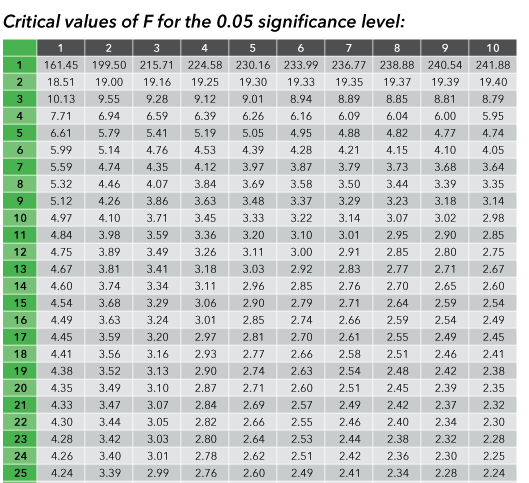12.07

Please click on the correct critical F value in the table, for the specified df. Use an α=.05.

$df_{Between}$ = 4 $df_{within}$ = 20One-way ANOVA

Scientists specify the type of ANOVA being conducted in terms of the number of independent variables or factors in the research design. A one-way ANOVA, also called a one-factor ANOVA, is another name for an ANOVA for a study with only one independent variable. Often, statisticians will include the number of levels of the independent variable (IV) in the label as well. For example, suppose we wanted to study the effect of a mnemonic technique on memory recall in children. We might assign children to one of three memory conditions: an imagery visualization group, a word repetition group, or a control no cue group. The dependent variable would be the number of words recalled on a memory task. The study design would be a one-factor, three-level design. The IV or factor is memory group, with three levels (visual imagery; repetition; control).

Statisticians also specify whether the participants were exposed to all levels or only one level of the independent variable.

Between-Groups Designs: Different participants receive each level of the independent variable (IV). That is, each participant receives only one level of the IV.

Within-Groups Designs: Each participant receives all levels of the independent variable. Within-groups designs are often referred to as repeated measures designs because each participant is repeatedly measured.

In our example above, each child received only one memory condition: imagery, repetition, or no cue. So the research design is a 1-factor, 3-level, between-groups design. Regardless of the type of ANOVA, the assumptions and interpretations are the same.

12.08

Match the research descriptions with the appropriate research design.

Premise
Response
1

Three groups of children were assigned to no reinforcement¸ an immediate reinforcement or delayed reinforcement reward group.

A

One-factor¸four-level¸between-groups

2

Patients response to a pain-management strategy was assessed before and after surgery.

B

One-factor¸three-level between-groups

3

Each of four brands of tires were tested.

C

One-factor¸two-level within-groups

Setting up the Null and Alternative Hypotheses

Recall the hypothesis testing steps:Figure 12.7: Steps in hypothesis testing​

Calculating the Sums of Squares

​You were introduced to the idea of using a sum of squares as an indicator of variability back in Chapter Two, Descriptive Statistics. The sum of squares represents a measure of variation or deviation from the mean, calculated as a summation of the squares of the differences from the mean. The calculation of the total sum of squares considers both the sum of squares from the factors being compared and from randomness or error.

In analysis of variance (ANOVA), the total sum of squares helps to express the total variation that can be attributed to various factors. For example, you do an experiment to test the effectiveness of three diet plans, measuring mean weight loss over a period of time. The total sum of squares (SStotal) = treatment sum of squares, shown as the sum of squares between treatment groups (SSbetween) + sum of squares of the residual error, shown as the sum of squares within treatment groups (SSwithin).

SStotal = SSbetween + SSwithin

The treatment sum of squares is the variation attributed to, or in this case between, the diet plans. The sum of squares of the residual error is the variation attributed to random error and individual differences within the treatment groups.

Converting the sum of squares into mean squares by dividing by the degrees of freedom lets you compare these ratios and determine whether there is a significant difference due to the treatment, i.e., the diet plan. The larger this ratio is, the more the treatments affect the outcome.Figure 12.8. Steps in computing ANOVA​

Figure 12.8 above outlines the steps required to conduct a one-way ANOVA.

Step 1: Compute the sums of squares.

In Step 1, the total sums of squares (SStotal) in the study is partitioned into the between-groups component of SS and the within-groups component of SS. The sum of both components is equal to the SStotal. Below are the computational formulas for the three types of Sums of Squares.

To compute the SStotal, treat the entire data set as if it were one large group. Note the similarity between the above SS formula and the formula we used to compute SS for t-tests.

SSwithin = ∑SSi

To compute the SSwithin, compute SS for each group individually, then add the separate Sums of Squares together. The ‘i’ is a placeholder for the number of groups in the study. For example, if the research design included three levels of the independent variable, then SSwithin would = SS1 + SS2 + SS3.

For a research design with two groups:

The number of terms in the computation for the SSbetween depends on the number of groups in the study. As in the formula for the SSwithin, the letter ‘i’ is used to denote the number of groups. For a research design with two groups or two levels of the independent variable, for each group first sum the scores, then square the sum of the x’s, then divide the value by the sample size for that group. Repeat this procedure for each group in the study. Add the values. The final term in the equation is subtracted. To compute the final term, add the individual sums of the scores (∑ x) for each group. Then, square the sum. Finally, divide the sum by the total sample size.

Step 2: Construct the source table.

The source table consists of the sources of variance (between, within, and total), the degrees of freedom, the sums of squares, the mean squares, the F-statistic, and the probability of the F

Step 3: Compute the degrees of freedom.

The dfbetween is equal to (the number of groups -1). The dfwithin is equal to (total sample size – the number of groups). The dftotal is the total number of data points – 1. Note that dfbetween + dfwithin = dftotal.

Step 4: Compute the mean square between-groups (MSbetween) and the mean square within-groups (MSwithin).
The mean square is an average sum of squares for each source of variance. To compute the mean of a sample, we divide the sum of all of the scores by n, the sample size. The logic of the mean square is similar to the logic of computing a mean. The mean square is the sum of squares (total variability) divided by the corresponding degrees of freedom (a measure of sample size).

To compute the MSbetween, divide the SSbetween by the df between

The MSbetween is our estimate of the between-groups variance.

To compute the MSwithin, divide the SSwithin by the dfwithin.

The MSwithin is the variance estimate for the variability within groups.

Step 5: Compute the F-statistic.

Recall that the F-statistic is a ratio of between-groups variance / within-groups variance. Our estimate of between-groups variance is the mean square between. Our estimate of within–groups variance is the mean square within. Therefore, the F statistic = MSbetween / MSwithin.

Step 6: Look up critical F and compare the computed F-statistic to critical F.

Now we are ready to evaluate whether the computed F-statistic exceeds the critical value of F. To look up the critical value of F, we need the appropriate degrees of freedom. The convention in the social sciences for writing the results of an ANOVA is as follows:

F (df numerator (between), df denominator (within)) = computed F-value, p = (probability level)

Step 7: Decide whether to reject or fail to reject H0.

If the computed F-ratio is smaller than the critical value of F, our decision must be to fail to reject H0. At the specified alpha level, the claim that there would be a significant treatment effect of the independent variable would not be supported. If the computed F-ratio is equal to or larger than the critical value of F, our decision would be to reject H0. The groups are not equal. The independent variable did have a significant effect.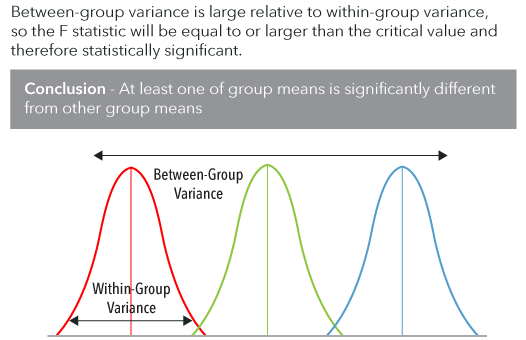Figure 12.10: Illustration of larger between-groups variance relative to within-groups variance

Step 8: If there are more than 3 levels of the IV and the decision is to reject H0, then compute the appropriate post-hoc test.

Post-hoc testing, which means “after the fact” testing, is computed only when the statistical decision is to reject H0 and only when additional testing is needed to discern group differences. Post-hoc testing involves all possible pair-wise comparisons of group means to discern which groups differ from each other.

Step 9: Interpret the results of the post-hoc test.

If the overall F-ratio was significant, then at least one pair-wise post-hoc comparison must exceed the critical value for that statistic. As with t-scores and F-scores, there are standard tables of critical values for post-hoc tests. Look up the critical value for the post-hoc test; if the computed value of the post-hoc statistic exceeds the critical value, then the two groups being compared are statistically significantly different.

Understanding and Calculating Effect Size: R2

Effect size calculations take hypothesis testing to a higher level. The probability of our test statistic indicates the likelihood by chance of obtaining an F-statistic of a given magnitude. Effect size is a measure of the proportion of variance in the dependent variable that is accounted for by the independent variable. One measure of effect size that is commonly used with ANOVA is R2 (read as R squared). The formula for R2 is

There are conventions for determining the magnitude of effect size: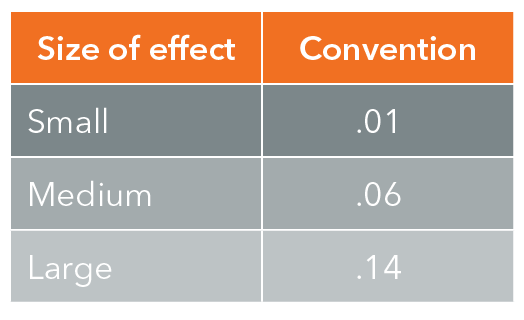Figure 12.11: Conventions for effect sizes (R2)

The R2 value provides information on the magnitude of a significant treatment effect. The P-value provides simply the likelihood of the treatment effect by chance.

12.09

Match the formula with the appropriate term.

Premise
Response
1

SS$_{total}$

A

$\frac {MS_{between}}{MS_{within}}$

2

SS$_{between}$

B

$∑x^2_{total} - \frac{∑(x_{total})^2}{N_{total}}$

3

SS$_{within}$

C

$\frac {SS}{df}$

4

MS

D

∑SS$_i$

5

$F$

E

$\frac{∑x_{i}^2}{N_i}-\frac{[∑x_i]^2}{N_{total}}$

ANOVA Example

We will follow the above steps for ANOVA with hypothetical data. Let’s suppose that you are conducting a study to evaluate the efficacy of requiring health care workers in low-income clinics to wear surgical masks for the reduction of tuberculosis (TB) cases among health-care workers. The study utilizes a one-factor, two-level between-groups design. Health care clinics in low–income areas are randomly assigned to the mandatory mask group or a control group. The number of medical staff cases of TB positive tests per clinic will be assessed. Given the nature and costs of the medical supplies, the researchers choose a .01 α level of significance. The researchers claim that there will be a significant difference in the number of new TB positive tests between the two clinic protocol groups. Just as for a two-sample t-test, H0 states that the mean number of TB cases for the two groups will not differ. H1 states that the mean number of TB cases for the two groups will not be the same. Rather than testing mean differences as in the t-test, we will use the F-test to evaluate the between-groups variance relative to the within-groups variance, a direct measure of the effect of the independent variable on the dependent variable.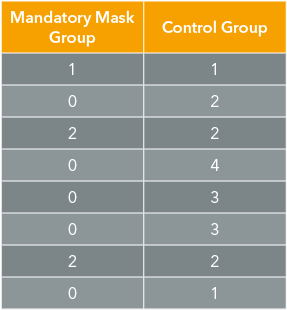Figure 12.12: Hypothetical data for our medical masks to prevent TB transmission study. The values represent the number of positive Staff TB tests.​

Write down the sample size for each group. Compute the mean and sums of squares for each group.

Step 1: Compute the sum of squares between (SSbetween), the sum of squares within groups (SSwithin) and the Sum of Squares total (SStotal)

The formula for the SStotal is:

Let’s look carefully at this formula. To compute the SStotal, treat the data as if all of the data points were in one large group. Let’s look at our table with our intermediate calculations: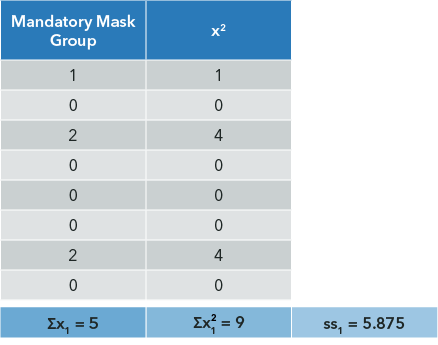Figure 12.14: Illustration of calculations for SS1Figure 12.15: Illustration of calculations for SS2

Plug the above values into the SStotal formula:

Note that SSwithin + SSbetween = SStotal

Step 2: Construct the source table.

The source table consists of the sources of variance (between, within, and total), the degrees of freedom, the sums of squares, the mean squares, the F-statistic, and the probability of the F. Fill in the SS values that we computed above.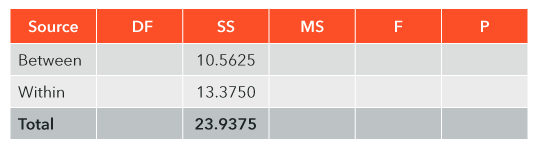Figure 12.16:​ Source table for the medical mask study​

Step 3: Compute the degrees of freedom.

The df between groups is equal to (the number of groups - 1). Since there are two groups in our study, the dfbetween is (2-1) or 1.

The dfwithin is equal to (total sample size – the number of groups). We have 16 clinics in our study and 2 groups. Therefore the dfwithin = (16 – 2) or 14.

The dftotal is the total number of data points – 1. There are 16 data points and 16-1 = 15. Note that dfbetween + dfwithin = dftotal.

Now that we have computed all of the degrees of freedom, let’s plug them into our source table.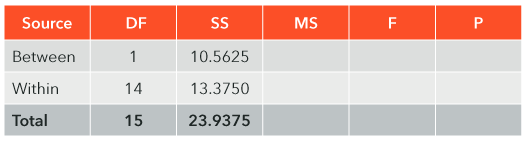Figure 12.17:​ Addition of the SS and df to the source table​

Step 4: Compute the mean square between groups (MSbetween) and the mean square within groups (MSwithin).

The mean square is an average sum of squares for each source of variance. To compute the mean of a sample, we divide the sum of all of the scores by n, the sample size. The logic of the mean square is similar to the logic of computing a mean. The mean square is the sum of squares (total variability) divided by the corresponding degrees of freedom (a measure of sample size).

To compute the MSbetween, divide the SSbetween by the dfbetween

The MSbetween is our estimate of the between-groups variance.

To compute the MSwithin, divide the SSwithin by the dfwithin.

The MSwithin is the variance estimate for the variability within groups.

Let’s add the MS values to our source table.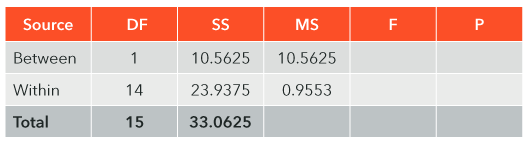Figure 12.18: Calculation of the mean square​​

Step 5: Compute the F-statistic.

Recall that the F-statistic is a ratio of between-groups variance/within-groups variance. Our estimate of between-groups variance is the mean square between. Our estimate of within-group variance is the mean square within. Therefore, the F-statistic = MSbetween / MSwithin

= 10.5625 / 0.9553

F = 11.06

Let’s fill in the F-value in our source table.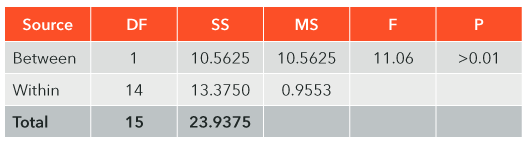Figure 12.19:​ Calculation and evaluation of the F ratio​

Step 6: Look up critical F and compare the computed F-statistic to critical F.

Now we are ready to evaluate whether the computed F-statistic exceeds the critical value of F. To look up the critical value of F, we need the appropriate degrees of freedom. The convention in the social sciences for writing the results of an ANOVA is as follows:

F (df numerator (between), df denominator (within)) = computed F-value, p = (probability level)

For our example:

F (1, 14) = 6.18, p = _____

In order to determine the probability of our F-score, we need to look up critical F. Remember that we used an α level set at .01.

Use the F-table to locate the critical value of F.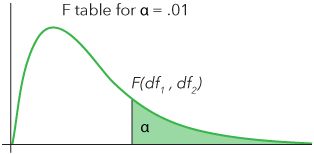Figure 12.20: Graphical representation of the critical F-value​​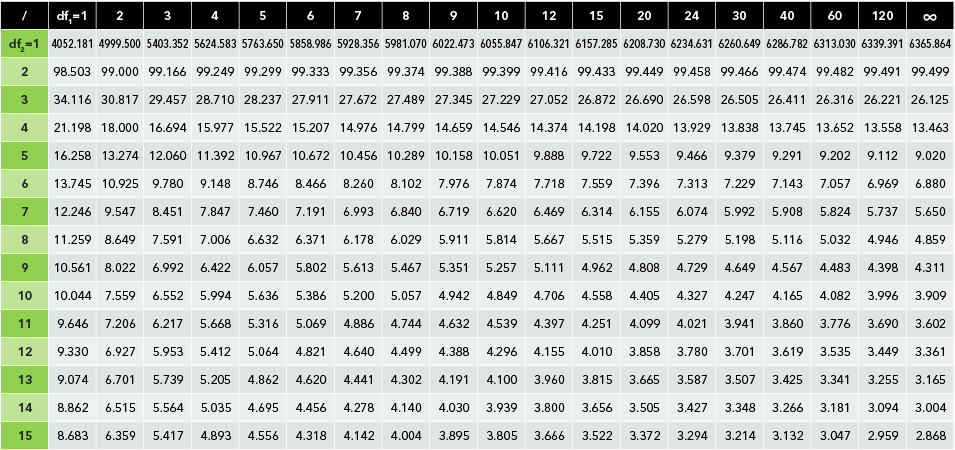Figure 12.21: F Table for ɑ=0.01

The critical value of F from the table is 8.862. Our computed F of 11.06 is larger than the critical F of 8.862.

Step 7: Decide whether to reject or fail to reject H0.

Since the computed F-ratio is larger than the critical value of F, our decision must be to reject H0. At the specified alpha level, the claim that there would be a significant difference in the number of new TB positive tests between the two clinic protocol groups is supported.

12.10

Let’s practice filling in missing values on the ANOVA source table. Assume an α level of .05 to evaluate the significance of F. What is the MS$_{within}$?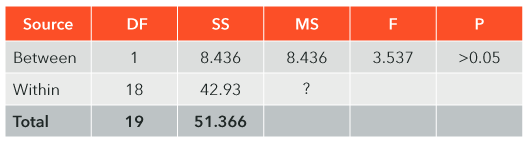Three-sample ANOVA

Note that in the one-way ANOVA formula there is no place for sample means. The formulas are identical whether there are 2, 3, or 23 levels of the independent variable. However, the one-way ANOVA tests the null hypothesis that all of the sample means are equal to each other. If there are only two groups and the decision is to reject H0, it is obvious which group scored higher or lower. However, with three or more groups in the analysis and H0 rejected, additional testing would be needed to determine which groups differed from each other. The same one-way ANOVA formulas apply. Let’s work through an example with three groups.

Example of Three-Group ANOVA

Suppose you need to buy new tires for your car and you want to get the best quality tires that you can. Suppose independent researchers recently tested three popular brands of tires: Michelin, Dunlop, and Firestone, each on five different vehicles. The data below are the number of months of routine daily driving during which each type of tire showed no signs of wear or defect. That is, the higher the score, the better the tire’s performance. Which brand of tire performed the best on the road test? Set the alpha level at .05.

Let’s start by identifying the design. There is one independent variable, the brand of tire, with three levels: Michelin, Dunlop, and Firestone. The dependent variable is months of driving without wear. Each of the vehicles tested was equipped with only one brand of tire, not all three. So, the independent variable, tire brand, is a between-groups variable. Therefore the research design is a 1 factor, 3 level, between-groups design. Since the dependent variable is a ratio-scaled variable, ANOVA is the appropriate statistical test to use.

Set up the appropriate hypotheses. The research claim is that all three brands of tires will not perform equally in the driving test. H0 states that the mean number of months without wear and tear will be the same for all three tire brands. H1 states that the mean number of months without wear and tear for all three tire brands will not be the same.

Just as in the two-group example, write down the sample size for each group. Compute the Mean and Sums of Squares for each group.

Michelin: 58, 60, 48, 52, 51

Dunlop: 36, 40, 42, 48, 38

Firestone: 32, 36, 42, 48, 40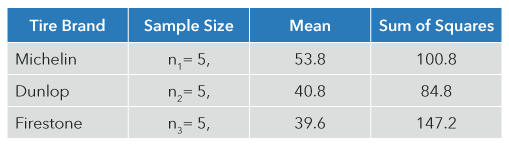Figure 12.22: Descriptive statistics for tire study​

Step 1: Compute the sum of squares between groups (SSbetween), the sum of squares within-groups (SSwithin) and the sum of squares total (SStotal)

Let’s begin with the SSwithin, which is the sum of the individual SSs for each tire.

To Compute the SStotal, treat all of the data as if it were one large group.

Since SSbetween + SSwithin = SStotal, we can simply subtract SSwithin from SStotal to determine

SSbetween

SSbetween = SStotal - SSwithin

SSbetween = 952.93 – 332.8

SSbetween = 620.13

Step 2: Construct the source table.

Fill in the sources of variance and the sums of squares. As you compute each of the next steps, fill in the ANOVA table.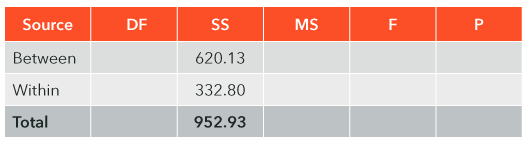Figure 12.23: Source table for the tire study​

Step 3: Compute the degrees of freedom.

dfbetween = # of groups – 1

Since there are 3 types of tires,

dfbetween = 3-1

dfbetween = 2

dfwithin = # of data points – number of groups (k)

dfwithin = 15 – 3

dfwithin = 12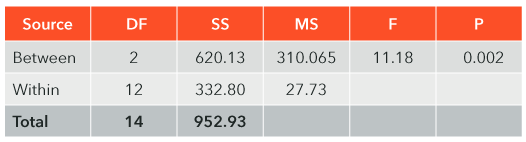Figure 12.24: Calculation and evaluation of the F-ratio for the tire study​

Step 4: Compute the mean square between groups (MSbetween) and the mean square within groups (MSwithin).

Step 5: Compute the F-statistic

Write the results in standard APA format: F (2, 12) = 11.18

Step 6: Look up critical F and compare the computed F-statistic to critical F.

We can see below in the excerpt from the F-table for α = .05, that critical F (2, 12) = 3.89. In fact, the P-value of our computed F is equal to 0.002. Our computed F-statistic definitely exceeds critical F from the table.Figure 12.25: Critical values of F​​

Step 7: Decide whether to reject or fail to reject H0.

Since the computed F of 11.18 exceeds the critical F of 3.89, our decision is to reject H0. The three brands of tires are in fact not equal in the number of months of normal driving before they show any wear. If our decision had been to fail to reject H0, we would be done with our analysis at this point. However, since our decision is to reject H0 and we know that the three tire brands are not equal, we need some additional testing to determine which tire brand differs from which.

Computation of R2

Since there is a significant effect of our independent variable (the type of tire) on the dependent variable (tread wear), we can compute R2 to determine the magnitude of the effect size.

According to convention, the effect size of tire brand on tread wear is quite large, since our computed effect size of .65 exceeds the conventional large effect size of .14. We can interpret R2 as such: 65% of the variance in tread wear is due to the brand of tire used.

Post-hoc Testing for Three or More Samples

Post-hoc testing, which means “after the fact” testing, is computed only when the statistical decision is to reject H0 and only when additional testing is needed to discern group differences. There is a collection of post-hoc tests. One that is routinely used for one-way ANOVA post-hoc comparison is known as the Tukey HSD test. HSD stands for honestly significant difference. The Tukey HSD considers all possible pairwise group comparisons and evaluates which pairs are honestly significantly different. In order to compute the HSD statistic, you will need the mean of each group (which we already computed above in Figure 12.21) and the standard error of the mean(SM, when the sample size for each of the groups is the same (as is the case in our example),

Step 8: If there are three or more levels of the IV and the decision is to reject H0, then compute the appropriate post-hoc test.

The HSD is computed for all possible pairs. In other words, we need to compute the mean difference in months of driving without tread wear between Michelin and Dunlop, between Dunlop and Firestone, and between Michelin and Firestone. In each case, we will divide the mean difference by the SM. If the resulting HSD statistic exceeds the critical HSD table value, then those two groups (or tire brands) are honestly statistically significantly different from each other.

Using the means from Figure 12.21, compute the mean differences between each pair of tire brands. Then divide the difference by the SM to determine the pairwise HSD.

Now that we have computed Tukey HSD values for all possible pairwise comparisons of tire brands, we need to consult a table of critical values of the HSD statistic to determine which pairs differ significantly. Since our F-statistic was significant, at least one of the pairs must be significantly different on the post-hoc Tukey HSD test, which is also known as a q-statistic. We need three pieces of information to look up the critical HSD value: the alpha level for the post-hoc test, the df within groups (also known as the Error Term), and the number of levels of the IV or treatment groups, designated as k in the table.

For our example, we will choose an α level of .05. (Note: the choice of α level does not need to be the same as the one used in the F-test.) The dfwithin can be found in the ANOVA source table; dfwithin = 12. The number of groups in our study or k=3, since we have three brands of tires.

In the table below, note the circled critical HSD values of 3.77 (α=.05) and 5.05 (α=.01). Any pairwise HSD values that we computed that exceed the critical HSD of 3.77 are significantly different at the .05 level. Any pairwise HSD values that exceed the critical HSD of 5.05 are significantly different at the .01 level.

Step 9: Interpret the results of the post-hoc test.

Two of our HSD values exceed the critical HSD: Michelin vs. Dunlop and Michelin vs. Firestone. Both are significant at the .01 level which exceeds the set alpha level of .05. In order to interpret the results, we need to look at the mean number of months without wear and tear on the tires. With a mean number of months of 53.8, Michelin tires performed best in the road test. The HSD test revealed that Michelin tires performed significantly better than Dunlop tires (HSD = 9.56, p<.01). Michelin tires performed significantly better than Firestone tires (HSD = 10.44, p<.01). Dunlop and Firestone tires did not differ significantly from each other, because the pairwise comparison HSD did not exceed the critical value. Our conclusion, therefore, is that all three brands of tires are not equivalent. Michelin tires performed significantly better than both Dunlop and Firestone tires.

Recap of When and Why to Include a Post-hoc Test

Post-hoc tests are used only after the initial overall F-statistic is significant. Following a significant F-test, at least one of the post-hoc tests must be significant. Post-hoc tests inform researchers which specific groups differ from each other by evaluating all possible pairwise comparisons of means.

12.11

Look up Tukey HSD critical values for the following research designs, α=.05.

A three-group, one-factor ANOVA with $df_{total}$= 20

12.12

Look up Tukey HSD critical values for the following research designs, α=.05.

A four-group, one-factor ANOVA with $df_{within}$ = 10

The Logic and Interpretation of Within-Groups ANOVA

Earlier in this chapter, you learned how to conduct a one-factor between-groups ANOVA. The three-group between-groups ANOVA is the multiple group equivalent of an independent t-test. In this section, we will learn the rationale and interpretation of a one-factor within-groups ANOVA. The within-groups ANOVA is the multiple group equivalent of the correlated or dependent t-test. Have you ever comparison shopped for the lowest price on college textbooks? If so, then you participated in a within-groups activity. You compared the price of the same textbook from multiple vendors.

The Benefits of a Within-Groups Design

In a within-groups design, all participants receive all levels of the independent variable (IV). Since the same participants are exposed to all levels of the IV, the variance due to individual differences, also known as error variance, is reduced. Practically speaking, each person brings his or her unique individual differences to each level of the IV. For example, if a make-up company wanted to test customer satisfaction for a new brand of mascara, asking all participants to try both the standard brand and the new brand of mascara would involve a within-groups design.

Another benefit of a within-groups design is the evaluation of an additional source of variance: the Participants (also known as Subjects). The Participants' source of variance allows researchers to evaluate and remove participant differences from the SSwithin, the estimate of variability across conditions. In other words, the Participants' SS will be subtracted from the within-groups SS, which will reduce the error variance in our ANOVA test. Reducing error variance (SSwithin) will reduce the denominator in the F-ratio, and is likely to lead to a significant F-ratio. However, degrees of freedom are computed a little differently in within-groups designs. The same participants are included at each level of the IV, but we count them only once. So the dfparticipants = the number of participants – 1; the dfwithin = (dfbetween) (dfparticipants). The resulting dfwithin is smaller than it would be had the same number of data points been collected in a between-groups design.

The Source of Variance and F-Ratio's in a One-Factor, Three-Group Within-Group ANOVA

Let’s consider an example in which a professor wants to evaluate the efficacy of allowing students to retake an online quiz in Statistics three times to maximize learning. The professor’s policy is such that online quizzes are available for 10 days; students are allowed to retake the quizzes three times, and the highest score achieved will become part of the course grade. The professor’s rationale is that students are given feedback on their scores each time they complete the quiz, so they know the questions and which ones they answered incorrectly. Therefore, the students can study the material that they got wrong and try again. The professor hypothesizes that the scores on subsequent attempts will be significantly higher than the scores on the previous attempts.

Figure 12.28​ below contains fictitious quiz scores for 10 students. The independent variable is the quiz attempt with three levels; the dependent variable is the score on the quiz. Since each student took the quiz three times, quiz attempt is a within-groups variable. The design of this experiment is a one-factor, three-level within-groups design. This is a good place to pause and think about the design description you just read. It is important to understand how the design of the quiz study differs from a between-groups design. You may want to go back and re-read this section.Figure 12.28: Fictitious quiz data in a within-groups experiment

The hypothesis-testing steps for the within-groups ANOVA are identical to those in Figure 12.8 for the between-groups ANOVA. The computational steps are quite similar to the steps outlined previously, with the exception of one additional source of variance: participants. The purpose of this section is to teach you how to identify research designs, choose the appropriate ANOVA, and interpret the output. In actual practice, most researchers do not compute F-statistics by hand. Rather, they use statistical software packages and interpret the output. Choosing the correct analysis from a variety of menu options is critically important, as is appropriate interpretation of the results. Let’s use the hypothetical quizzing data to practice interpreting the results. Below are summary statistics, the ANOVA Source Table, and the output of post-hoc pairwise comparisons for the quizzing data—the type of information that would be in a standard output file from a computerized statistical software package:Figure 12.29. Descriptive statistics for the hypothetical quizzing study​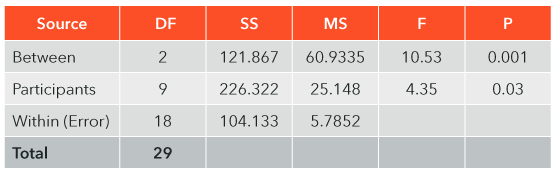Figure 12.30. ANOVA table for hypothetical quizzing study​​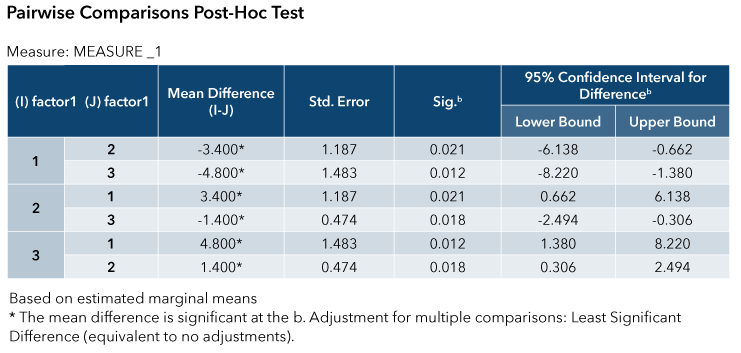Figure 12.31: Post-hoc pairwise comparisons for the hypothetical quizzing study​​

Extracting the Results from the Statistical Output and Summarizing the Findings

Looking at the ANOVA table for the between source of variance, which is the quiz attempts, we see that F (2, 18) = 10.53, p=.001. Since the probability of the F-score is less than .05, our decision is to reject H0. The three-attempt mean quiz scores are not all the same. Since there are three groups, we do not know which differs from which; just as we did in the between-groups three-group ANOVA previously, we will need to conduct a post-hoc test. Post-hoc tests can be chosen from a menu in most computerized statistical packages. Figure 12.31 contains the output of post-hoc pairwise comparisons. All pairwise comparisons of group means were significantly different at the .01 or .02 probability level. Since all differences exceed the alpha level of .05, all three-attempt mean scores differ significantly from each other. Look at the mean scores in Figure 12.30. The professor must conclude that the students performed significantly better on each quiz than on the previous one. It seems that providing students with three opportunities to retake the online quizzes results in statistically significant improvement in quiz scores.

The raw data for the between-groups tire study and the within-groups online quizzing study are provided so that you can practice entering data into a statistical software package, choosing the appropriate analyses, and interpreting the results.

12.13

In a within-groups design, which of the following is true?

A

Each participant receives only one level of the independent variable.

B

Each participant receives all levels of the independent variable.

C

Only some of the participants are tested more than once.

12.14

Which source of variance is found in a within-groups design, but not in a between-groups design?

A

Error variance

B

Between

C

Within

D

Participants

12.15

Which of the following is a benefit of a within-groups design over a between-groups design?

A

The variance due to individual differences is reduced in the within-groups design

B

The error degrees of freedom increases in the within-groups design

C

The alpha level is automatically reduced in a within-groups design

D

Fewer participants are needed in a between-groups design

Two-Way ANOVA – Factorial Designs

Rationale for Factorial Designs

In our examples above of one-way ANOVA, there was one independent variable and one dependent variable in each study design. In actual practice, researchers often test the effects of more than one independent variable on the same dependent variable. When more than one independent variable is included in a study, the design is known as a factorial design. In addition to evaluating the effects of each IV on the DV, factorial designs allow scientists to investigate interaction effects between two or more Independent variables.

The number of independent variables in the study determines the type of factorial ANOVA computed. The number of factors is the number of IVs. Just as for a one-way ANOVA, the dependent variable must be measured on an interval or ratio scale of measurement. In psychology, the most common type of research design is the 2 × 2 factorial design. Factorial research designs decrease within-groups variability, increase measurement sensitivity, better represent real-world phenomena, and increase the generalizability of findings. Studying the effects of single independent variables in isolation reveals nothing about the interdependence or interaction between independent variables. Only factorial designs can assess interaction effects between independent variables.

Factorial ANOVA Source Table Interpretation

Computing the variance components for factorial ANOVAs by hand is long and cumbersome. In actual practice, scientists use computerized statistical packages to compute the sums of squares, mean squares, F-statistics, and probabilities for each main effect and the interaction effect. Regardless of whether the components are computed by hand or by computer, you will need to understand how to interpret the factorial ANOVA source table. Just as in the one-way ANOVA, each independent variable is a source of variance, with degrees of freedom, sums of squares, and mean squares as well as an F-value and a probability value. However, the factorial ANOVA source table includes an additional source of variance: the interaction between the independent variables. If there is a significant interaction effect, the main effects of each independent variable change when independent variables are combined.Figure 12.32: Sample 2X2 ANOVA source table​​

Example of a 2X2 ANOVA: Decription, Hypotheses, Source Table, and F-statistic Interpretation

Suppose we conducted a 2 × 2 (Gender × Drug) study on the effectiveness of two well-known medications for treating blood clots: Aspirin and Heparin. Both IVs are between groups IVs; the DV is the percent of improvement in the number of blood clots following 6-weeks of treatment. There were 50 males and 50 females in the study. Twenty-five of each gender were treated with Aspirin; the other 25 of each gender were treated with Heparin. Figure 12.33 summarizes the study design. A 2 × 2 (Gender × Drug) between-groups design results in the following 4 groups:

The following results are hypothetical mean percentages of improvement. The ANOVA source table includes three separate F-tests: an F-statistic to evaluate the effect of the drug on blood clot reduction, an F-statistic to evaluate gender differences on blood clot reduction, and an F-statistic to evaluate any interaction effects between drug treatment and gender. The analysis reveals main effects for each independent variable, as well as any interaction effects. The researchers specify a separate null and alternative hypothesis for each independent variable. In addition, researchers specify null and alternative hypotheses for the interaction between the two independent variables. If there is a significant interaction effect, the main effects change when independent variables are combined.

Let’s look at the table of means for each independent variable for our hypothetical data:Figure 12.34: Table of means for the main effect of each independent variable​

The above table reveals that males scored much higher than females on the percent improvement measure. For the drug treatment, there does not appear to be much of a difference between the two treatments: Aspirin (mean score = 47.5) and Heparin (mean score = 50).

Now let’s look at Figure 12.35, which includes the means for all possible combined levels of the two independent variables. The means in the margins of the table are identical to the main effect means listed above in Figure 12.34. The marginal means are the mean scores for each level of each IV considered independently of any other IVs. In other words, the means for males and females are the average across both drug groups. Likewise, the means for each drug treatment groups are averaged across the two genders.

The four numbers inside the table are the means for the combined levels of the two independent variables, used to evaluate possible interaction effects. [Note: think ‘M’ for ‘margins’ and ‘main effects. Think ‘I’ for ‘inside’ and ‘interaction effects’.] The main effects indicated that males fared better than females overall, and that the effects of Aspirin and Heparin did not differ. However, when the two IVs are combined, the interaction effect looks quite different. The results reveal that males respond better to Heparin than Aspirin, but females respond better to Aspirin than Heparin. The main effect ‘changes’ when two or more IVs are combined, and the resulting effect is called an interaction effect. Spend some time carefully reviewing the data in Figures 12.34 and 12.35. Can you summarize the findings in your own words? Your summary should match the description of the main effects and interaction described earlier in this paragraph.Figure 12.35: Main effects and interaction means for a 2X2 between-groups ANOVA​​

Let’s look at the ANOVA source table for our hypothetical 2 × 2 ANOVA. The sources of variance are determined in the same way as for a one-way ANOVA. For each IV we will compute df, SS, MS, and an F-statistic. Note that there are three separate F-statistics: one for each IV, and a third for the interaction effect.

The df for each IV source of variance is computed as the number of groups (k) – 1.

The df for the within-groups variance or error variance is the number of data points or sample size minus the number of groups, (N-k).

dfwithin = 100 - 4

dfwithin = 96

The df for the interaction term is a new term for us. The degrees of freedom for the Interaction are computed as the product of the dfs for each IV that comprises the interaction. Since the df for gender = 1 and the df for drug treatment = 1,

df for the gender × drug treatment Interaction = 1 × 1 = 1

The dftotal = the number of data points (or sample size) – 1 = 100 - 1

dftotal = 99Figure 12.36: The ANOVA source table​​

Look at the probabilities associated with each of the three F-statistics. The first F-statistic for gender has a probability level of .0001, which is highly significant. Since the computed F exceeds critical F, the researcher will reject the null hypothesis that there is no gender difference in treatment outcome. Since there are only two groups (male or female), no post-hoc testing is needed. Now look at the probability associated with the computed F-statistic for the effect of drug treatment (F=2.12, p = .12). The computed F does not exceed critical F. The researcher must fail to reject the null hypothesis. The percentage improvement does not differ between Aspirin and Heparin. The third F-statistic, which evaluates the interaction effect, is highly significant. Note that p = .0001 for the computed F-statistic of 180.7. The researcher must reject the null hypothesis that all four groups: males given Aspirin, males given Heparin, females given Aspirin, and females given Heparin are equal. Look at the inside values of Figure 12.35 and the graph of the four group means below. The interaction pattern reveals that males respond better to Heparin than Aspirin, but females respond better to Aspirin than Heparin. Had the two independent variables not been tested together in the same study, the interaction between gender and drug treatment would not have been uncovered. Interaction effects reveal combined effects between independent variables.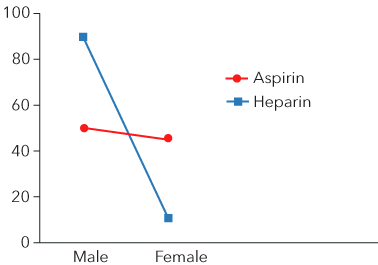Figure 12.37: Graph of the interaction between drug treatment and gender​​

Real-World Example of Factorial Designs and Interaction Effects

ANOVA Summary

In this chapter, we extended our data analysis capabilities beyond the two-group comparison. The F-statistic is used to compare two or more groups. Running multiple t-tests results in probability pyramiding, which increases the chance of Type I errors. ANOVA allows multiple comparisons with a single statistic: the F-statistic. We followed the same seven hypothesis-testing steps as in previous chapters. We expanded our interpretation of a significant effect to include post-hoc testing when appropriate as well as the computation of R2 as a measure of effect size (the proportion of variance in the dependent variable due to the independent variable).

This chapter provided an introduction to the computations and interpretation of one-way, between-groups ANOVA. Additionally, we explored the logic and interpretation of one-way within-groups ANOVA and 2 × 2 between-groups factorial ANOVA, including an explanation of interaction effects. There are additional types of ANOVA designs including mixed designs, which are beyond the scope of this chapter. Although the mathematical computations vary, the logic and interpretation of all ANOVA designs are the same.

You can experiment with the settings of the demonstration below to see how the results change.This Content is Locked
Only a limited preview of this text is available. You'll need to sign up to Top Hat, and be a verified professor to have full access to view and teach with the content.

Case Study: The Flipped Classroom Study

Davies, Dean, and Ball (2013) researched the efficacy of traditional classroom instruction, a flipped classroom, and computer simulations on learning outcomes in college students enrolled in an introductory course on spreadsheets. The goal of the study was to test for significant differences in the teaching approaches.

(Unfamiliar with flipped classrooms? Watch below for a short explanation.)

The researchers employed a 3 × 2 (teaching strategy × pre/post time) mixed design. The first independent variable was teaching strategy with three levels: traditional lecture, flipped classroom, or computer simulations. The second independent variable was time: before and after the teaching intervention. The dependent variable was test scores. Read through the study. Note the tables of means and Figure 12.6.

Case Study Question 12.01

Case Study Question 12.01

Are there significant main effects for each independent variable?

Click here to see the answer to Case Study Question 12.01.

Case Study Question 12.02

Case Study Question 12.02

Is there a significant interaction effect?

Click here to see the answer to Case Study Question 12.02.

Case Study Question 12.03

Case Study Question 12.03

Although the research design is a bit more complicated than the ones we worked within this chapter, you should have enough knowledge after reading this chapter to understand why ANOVA was the appropriate test statistic and the meaning of the results. Which is the best instructional method to use?

Click here to see the answer to Case Study Question 12.03.

Case Study Question 12.04

Case Study Question 12.04

Can you think of any additional independent variables that may influence the outcome of this study?

Click here to see the answer to Case Study Question 12.04.

References

R.S. Davies, D.L. Dean, Nick Ball (2013). "Flipping the classroom and instructional technology integration in a college-level information systems spreadsheet course," Educational Technology Research and Development (ETR&D), 61:4, pp. 563-580.

Pre-Class Discussion Questions

Class Discussion 12.01

Class Discussion 12.01

How does the F-distribution differ from the t-distributions?

Click here to see the answer to Class Discussion 12.01.

Class Discussion 12.02

Class Discussion 12.02

What is probability pyramiding?

Click here to see the answer to Class Discussion 12.02.

Class Discussion 12.03

Class Discussion 12.03

What does within-groups variance represent?

Click here to see the answer to Class Discussion 12.03.

Class Discussion 12.04

Class Discussion 12.04

Why is the F-statistic referred to as a ratio?

Click here to see the answer to Class Discussion 12.04.

Class Discussion 12.05

Class Discussion 12.05

When is a post-hoc test necessary?

Click here to see the answer to Class Discussion 12.05.This Content is Locked
Only a limited preview of this text is available. You'll need to sign up to Top Hat, and be a verified professor to have full access to view and teach with the content.

Answers to Case Study Questions

Answer to Case Study Question 12.01

There are no significant effects for type of instruction. There was a significant pre-test / post-test main effect. Scores on the post-test were significantly higher than scores on the pre-test.

Click here to return of Case Study Question 12.01.

Answer to Case Study Question 12.02

Yes. There was a significant method of instruction by time interaction. During the post-test period, scores in the excel simulation method were significantly lower than scores in the regular and flipped classroom conditions.

Click here to return of Case Study Question 12.02.

Answer to Case Study Question 12.03

Both the regular or flipped classroom instructional method were better than the Excel simulation method. The regular and flipped classroom methods did not differ from each other.

Click here to return of Case Study Question 12.03.

Answer to Case Study Question 12.04

Other possible variables that may influence the outcome of this study are: the level of technological experience of the students; the amount of experience with online learning; characteristics of the instructors; whether the course was required or an elective; and motivation of the students.

Click here to return of Case Study Question 12.04.

Answers to Pre-Class Discussion Questions

Answer to Class Discussion 12.01

Unlike the t-distributions, the F-distribution is a one-sided distribution, with only positive values. The shape resembles the right half of the standard-normal curve. Like the t- distribution, the F-distribution is a family of distributions; the critical values vary with the sample size. All F-scores are positive and two types of degrees of freedom are required to determine the critical value of F: the degrees of freedom between groups and the degrees of freedom within groups.

Answer to Class Discussion 12.02

Probability pyramiding is the inflation in Type I error rate due to the conduction of multiple statistical tests on the same data set.

Answer to Class Discussion 12.03

The within-groups variance reflects individual differences among the participants within each level of the independent variable. It does not reflect any effect of treatment, the independent variable.

Answer to Class Discussion 12.04

The F-statistic is computed as a ratio of between-group variance (which includes the effects of the independent variable and individual differences) divided by within-group variance (a separate measure of individual differences). The resulting one-number F statistic is a measure of treatment effect, or the effect of the independent variable on the dependent variable.

Answer to Class Discussion 12.05

A post-hoc test is necessary when the overall F-statistic is significant and there are three  or more groups, or levels of the independent variable.